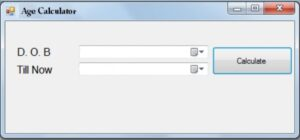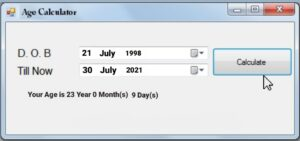# How to Calculate Age from DOB in VB.NET

Create a simple Visual basic application to display the current age from date of birth. In this C# code example, we will learn how to Calculate age till now from your given DOB(Date of Birth).

## Age calculator in VB.NET

Here in this program, I will explain how to calculate your current age by entering the Date of Birth and today’s date or we can also say that it is basically an age calculator.

For doing this calculation we have to take two date time picker and enter the date of birth and Current date respectively. For showing the result we have to take one label. In the label, we will display age in years with months and days. This works with a very simple logic we are doing this work in VB.NET.

#### Designing of the form:

This is a screenshot of the form design of the age calculator. Here I have taken 3 labels. I have named the first label as DOB and the second as a Current date and the last label is for showing the output so leave it empty also I have taken 2 date time picker and one button I have given the name of the button is Calculate. The date of birth and the current date as input and after the processing, the result will display. Here everything works in a single form.## Program to calculate age from DOB in VB.NET

```Public Class Form1
Dim datevalue1 As Date
Dim datevalue2 As Date
Dim datet As TimeSpan
Dim diff As Double

Private Sub btnCalc_Click(sender As Object, e As EventArgs) Handles btnCalc.Click
datevalue1 = Me.DateTimePicker1.Value.ToShortDateString
datevalue2 = Me.DateTimePicker2.Value.ToShortDateString
datet = (datevalue2 - datevalue1)
diff = datet.Days
Me.Label3.Text = ("Your Age is " + Str(Int(diff / 365)) + " Year")
diff = diff Mod 365
Me.Label3.Text = Me.Label3.Text + (Str(Int(diff / 30)) + " Month(s) ")
diff = diff Mod 30
Me.Label3.Text = Me.Label3.Text + (Str(diff) + " Day(s)")
End Sub
End Class```

#### Working of the code:

In the source code, four variables are created. Date of birth is stored in datevalue1 and the current date will be stores in datevalue2. After the subtraction, the result will stores in datet. Here 3 different formulas are used to calculate years, months, and days and the difference will be stored in diff variables. For calculating the years, months and days diff variables will be used in a different manner for each. While running the program the form will display and in the dob section, you have to choose the date of birth in the date time picker, and in the current date, you have to choose the current date or today’s date. Then the output is display in the third label in the form of years, months, and days.

#####So in this way, we can simply create an age calculator using VB.NET.